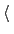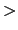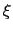Next: Probability distribution-restraints Up: Biasing and analysis methods Previous: Adaptive Linear Bias/Experiment Directed   Contents   Index

Subsections

## Multidimensional histograms

The histogram feature is used to record the distribution of a set of collective variables in the form of a N-dimensional histogram. It functions as a collective variable bias'', and is invoked by adding a histogram block to the Colvars configuration file.

As with any other biasing and analysis method, when a histogram is applied to an extended-system colvar (13.2.4), it accesses the value of the fictitious coordinate rather than that of the true'' colvar. A joint histogram of the true'' colvar and the fictitious coordinate may be obtained by specifying the colvar name twice in a row in the colvars parameter: the first instance will be understood as the true'' colvar, and the second, as the fictitious coordinate.

In addition to the common parameters name and colvars described above, a histogram block may define the following parameter:

• name: see definition of name (biasing and analysis methods)
• colvars: see definition of colvars (biasing and analysis methods)

• outputFreqFrequency (in timesteps) at which the histogram files are refreshedContext: histogram
Acceptable values: positive integer
Default value: colvarsRestartFrequency
Description: The histogram data are written to files at the given time interval. A value of 0 disables the creation of these files (note: all data to continue a simulation are still included in the state file).

• outputFileWrite the histogram to a fileContext: histogram
Acceptable values: UNIX filename
Default value: outputName.name.dat
Description: Name of the file containing histogram data (multicolumn format), which is written every outputFreq steps. For the special case of 2 variables, Gnuplot may be used to visualize this file.

• outputFileDXWrite the histogram to a fileContext: histogram
Acceptable values: UNIX filename
Default value: outputName.name.dat
Description: Name of the file containing histogram data (OpenDX format), which is written every outputFreq steps. For the special case of 3 variables, VMD may be used to visualize this file.

• gatherVectorColvarsTreat vector variables as multiple observations of a scalar variable?Context: histogram
Acceptable values: UNIX filename
Default value: off
Description: When this is set to on, the components of a multi-dimensional colvar (e.g. one based on cartesian, distancePairs, or a vector of scalar numbers given by scriptedFunction) are treated as multiple observations of a scalar variable. This results in the histogram being accumulated multiple times for each simulation step or iteration of cv update). When multiple vector variables are included in histogram, these must have the same length because their components are accumulated together. For example, if,andare three variables of dimensions 5, 5 and 1, respectively, for each iteration 5 triplets() are accumulated into a 3-dimensional histogram.

• weightsTreat vector variables as multiple observations of a scalar variable?Context: histogram
Acceptable values: list of space-separated decimals
Default value: all weights equal to 1
Description: When gatherVectorColvars is on, the components of each multi-dimensional colvar are accumulated with a different weight. For example, ifandare two distinct cartesian variables defined on the same group of atoms, the corresponding 2D histogram can be weighted on a per-atom basis: to compute an electron density map, it is possible to use weights [\$sel get atomicnumber] in the definition of histogram.

### Grid definition for multidimensional histograms

Like the ABF and metadynamics biases, histogram uses the parameters lowerBoundary, upperBoundary, and width to define its grid. These values can be overridden if a configuration block histogramGrid { ...} is provided inside the configuration of histogram. The options supported inside this configuration block are:

• lowerBoundariesLower boundaries of the gridContext: histogramGrid
Acceptable values: list of space-separated decimals
Description: This option defines the lower boundaries of the grid, overriding any values defined by the lowerBoundary keyword of each colvar. Note that when gatherVectorColvars is on, each vector variable is automatically treated as a scalar, and a single value should be provided for it.
• upperBoundaries: analogous to lowerBoundaries
• widths: analogous to lowerBoundariesNext: Probability distribution-restraints Up: Biasing and analysis methods Previous: Adaptive Linear Bias/Experiment Directed   Contents   Index
vmd@ks.uiuc.edu